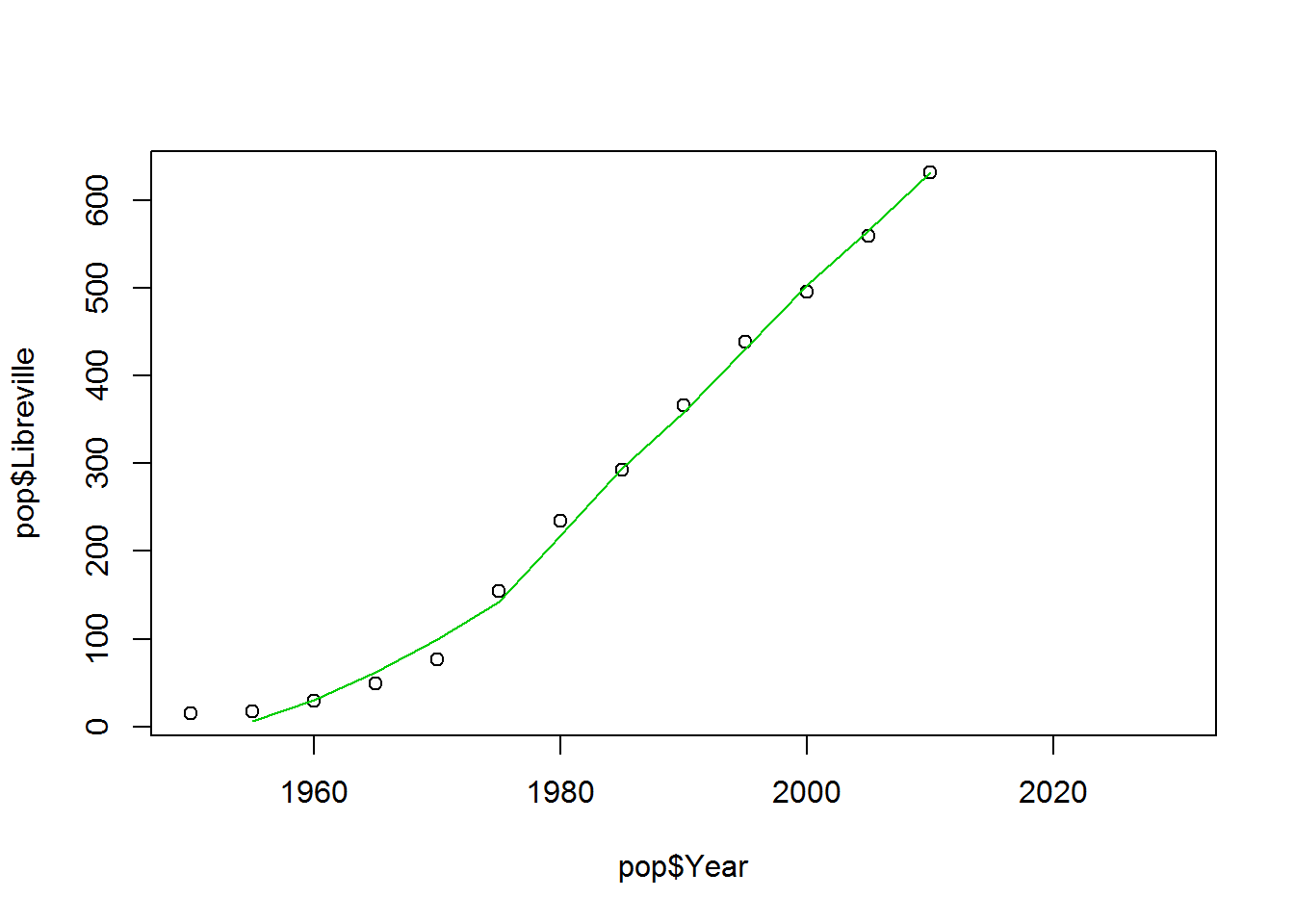# Planning Methods: Population trend model

Now that you have uploaded and examined the UN data on central African cities, we are going to do some ordinary least squares regressions (OLS) to predict Libreville’s population. For a review of OLS, please refer to class readings:

An Introduction to Statistical Learning, chapter 3

Video lectures available here (also, chapter 3)

Tufte’s Data Analysis for Politics and Policy (\$2 for ebook)

Let’s start by looking at the population over time:

``plot(pop\$Year, pop\$Libreville)``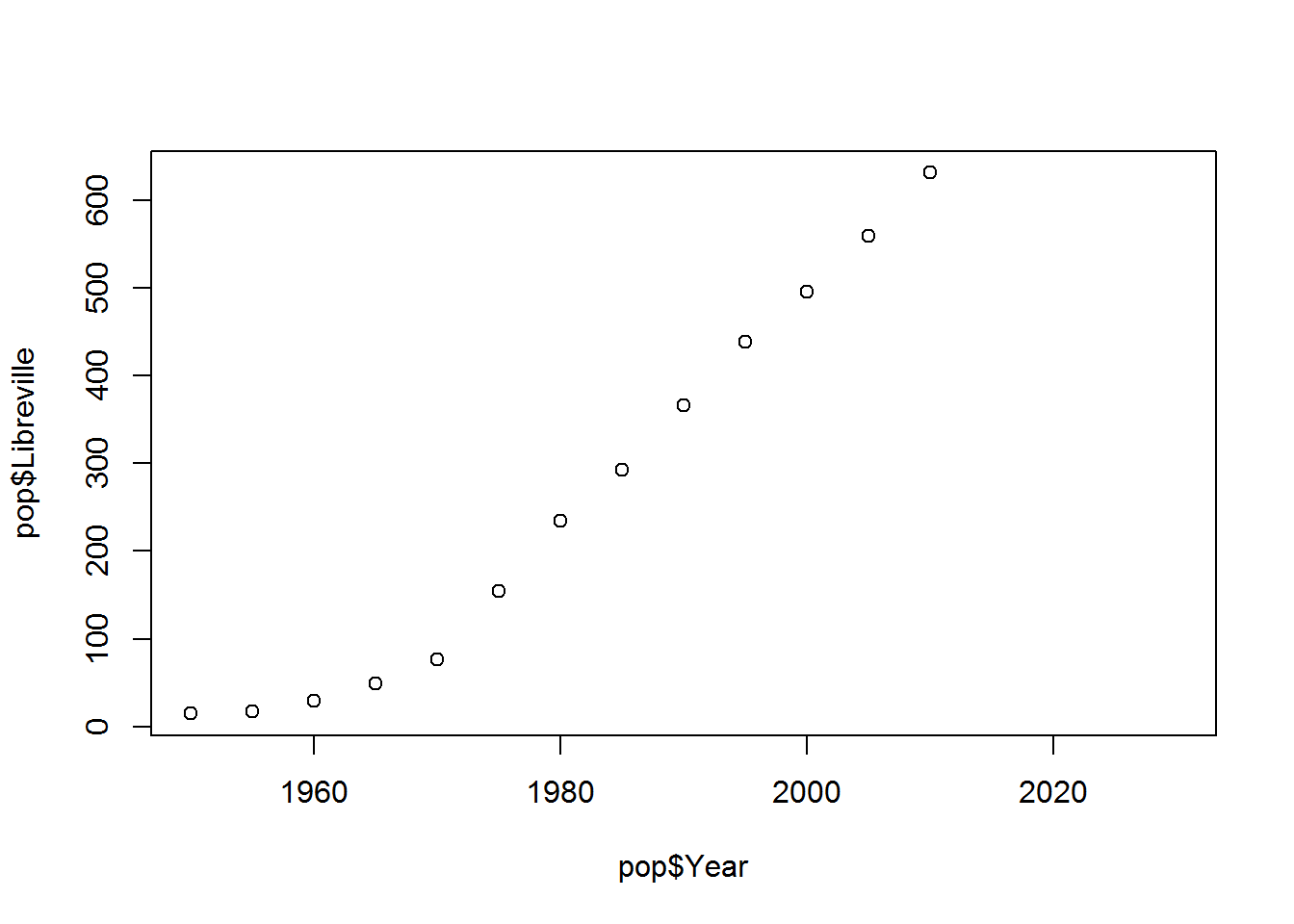It looks like we have a pretty linear trend from 1950 to 1975, with a steeper but still linear trend after 1975. If we predict the population growth by year using linear OLS and the full set of data, we’ll get a line that minimizes the squared distance between all the points in each year. In R, we can use the lm function (short for linear model) to estimate the slope and intercept of the line.

``````lbv <- lm(pop\$Libreville ~ pop\$Year)
summary(lbv)``````
``````##
## Call:
## lm(formula = pop\$Libreville ~ pop\$Year)
##
## Residuals:
##    Min     1Q Median     3Q    Max
## -69.81 -24.46  -4.11  21.42  91.48
##
## Coefficients:
##               Estimate Std. Error t value Pr(>|t|)
## (Intercept) -2.185e+04  1.314e+03  -16.62 3.84e-09 ***
## pop\$Year     1.116e+01  6.637e-01   16.82 3.39e-09 ***
## ---
## Signif. codes:  0 '***' 0.001 '**' 0.01 '*' 0.05 '.' 0.1 ' ' 1
##
## Residual standard error: 44.77 on 11 degrees of freedom
##   (4 observations deleted due to missingness)
## Multiple R-squared:  0.9626, Adjusted R-squared:  0.9592
## F-statistic:   283 on 1 and 11 DF,  p-value: 3.389e-09``````

What do the estimates mean?

The predicted population (the line that minimizes the squared distance between the points) is equal to the intercept plus the year times the estimated coefficent for year.

So if we want to know the estimated population in 2000, we could estimate it manually as -21848 + 2000 * 11.16. We can also use the outputs from the regression to save the trouble of entering the numbers manually.

``summary(lbv)\$coefficients + summary(lbv)\$coefficients*2000``
``##  481.7582``

Or we could predict each year in the data:

``summary(lbv)\$coefficients + summary(lbv)\$coefficients*pop\$Year``
``````##   -76.48352 -20.65934  35.16484  90.98901 146.81319 202.63736 258.46154
##   314.28571 370.10989 425.93407 481.75824 537.58242 593.40659 649.23077
##  705.05495 760.87912 816.70330``````

Or look at the predictions next to the data (note how I use the predict funtion instead of the manual estimation from above).

``cbind(pop\$Year, pop\$Libreville, predict(lbv, newdata = pop))#NB when you see a new command like cbind or predict, don't forget to learn more about it: ?cbind``
``````##    [,1] [,2]      [,3]
## 1  1950   15 -76.48352
## 2  1955   17 -20.65934
## 3  1960   29  35.16484
## 4  1965   49  90.98901
## 5  1970   77 146.81319
## 6  1975  155 202.63736
## 7  1980  234 258.46154
## 8  1985  293 314.28571
## 9  1990  366 370.10989
## 10 1995  439 425.93407
## 11 2000  496 481.75824
## 12 2005  559 537.58242
## 13 2010  631 593.40659
## 14 2015   NA 649.23077
## 15 2020   NA 705.05495
## 16 2025   NA 760.87912
## 17 2030   NA 816.70330``````

Is this a good predictor of population from 2015 to 2030?

The R2 from the regression indicates that our model explains 96% of the variation in population. That sounds pretty good, but is it really a good predictor of future population? To try to answer that question, let’s start by looking at the regression line?

``````plot(pop\$Year, pop\$Libreville)
abline(lbv)``````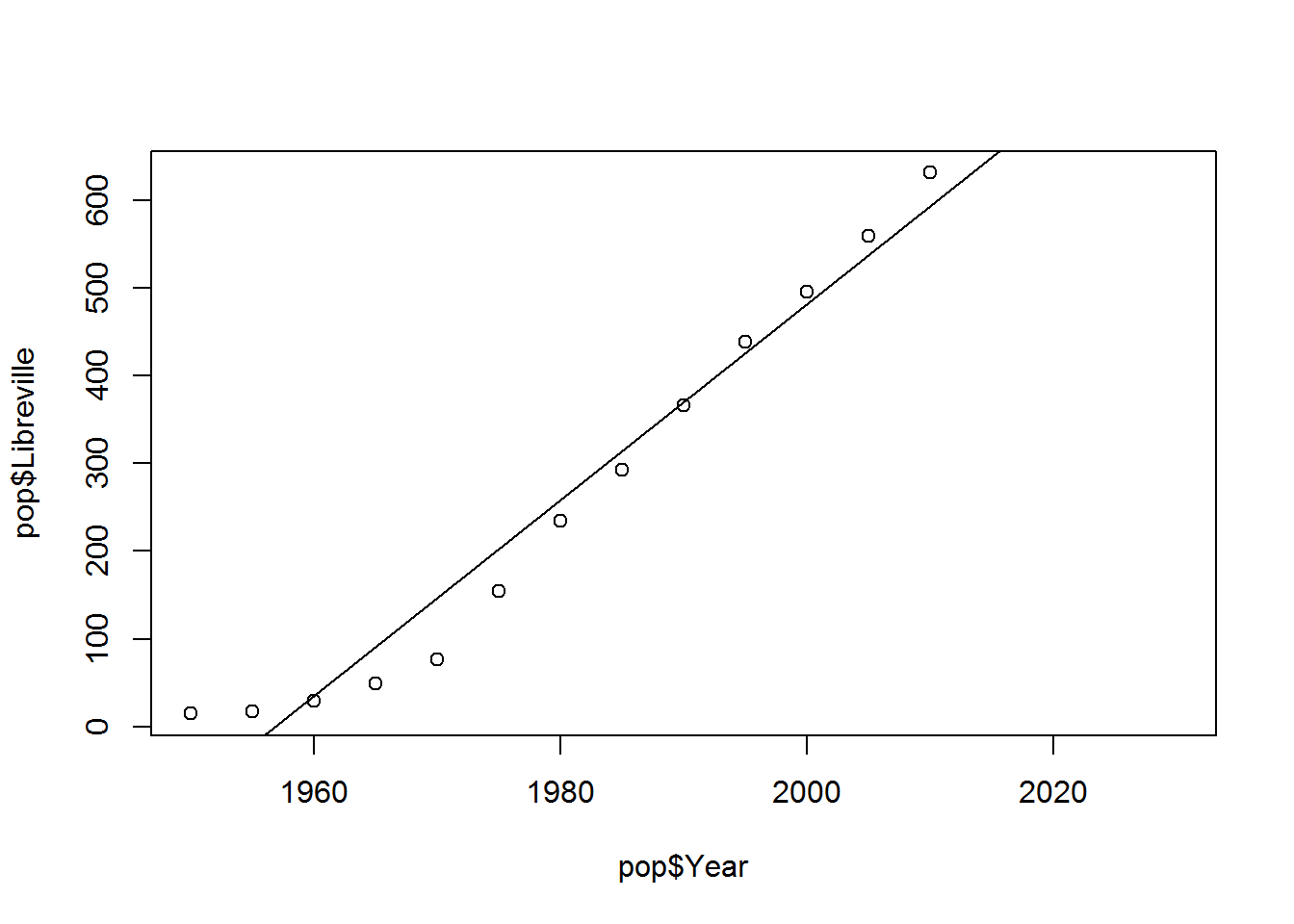The fit looks decent, but we also appear to be systematically underpredicting the population before 1960, overpredicting between 1965 and 1990, and underpredicting again after 1990. We also predict negative population before 1995. This is quite apparent, when we plot the predicted values against the error terms. (Remember that we want our error terms to be uncorrelated and normally distributed around a mean of zero.)

``````plot(predict(lbv), resid(lbv))
abline(h=0)``````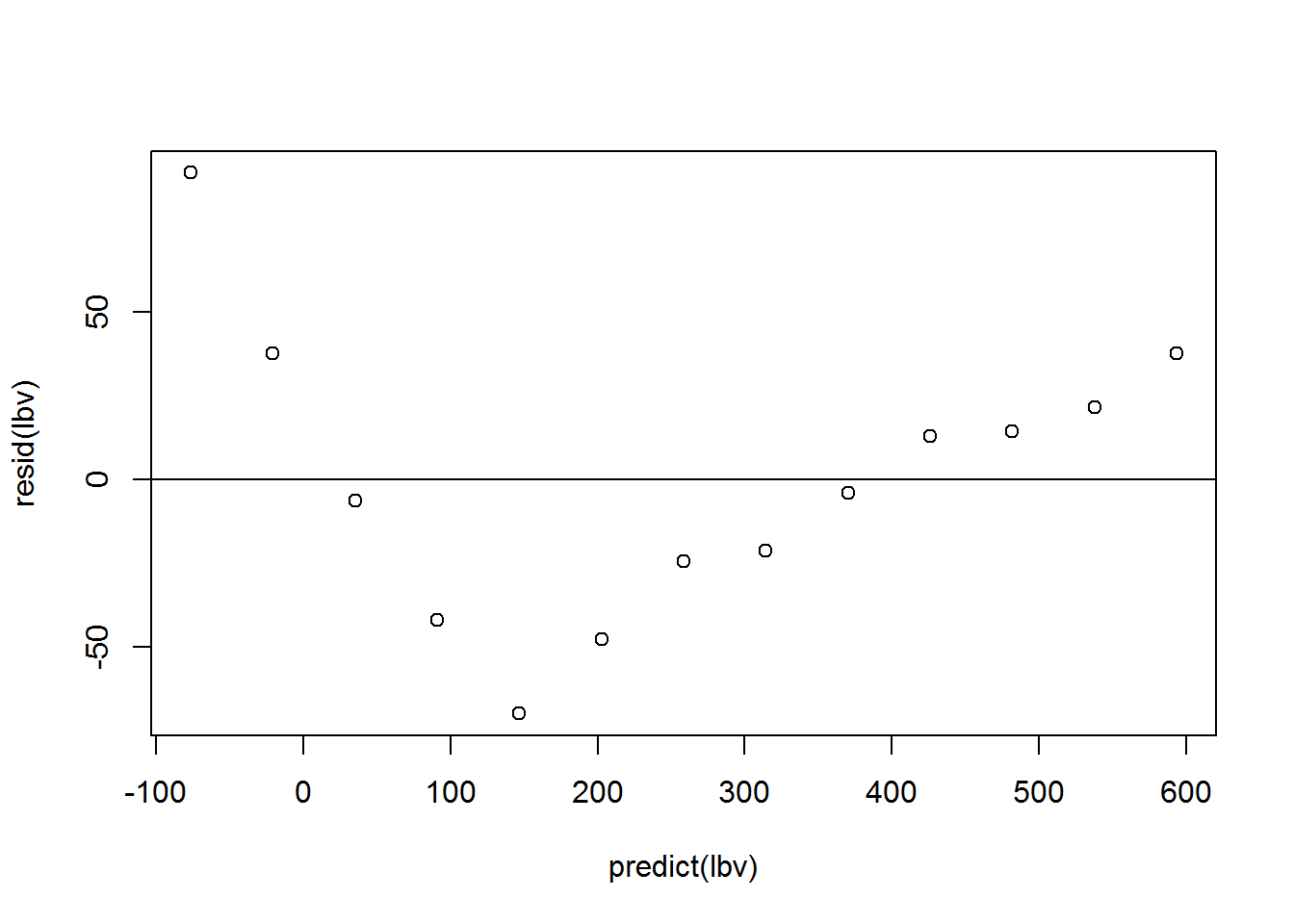Do you expcet a population prediction for 2020 based on the trend line from 1955 to 2010 to overestimate or underestimate population? Why?

If you guessed underpredict, then I agree. While we don’t necessarily know that the ongoing trend will continue, we’re making increasinly bad estimates from 1995 and on. If you knew Libreville, you would also probably not guess that population growth is going to slow in the near future. *****

Another way that we might test our model is to see how well our general modeling framework predicts values outside of the sample used in the regression. For cross-sectional, we might fit our model on a random sample of our data and then see how well it predicts other data.

Since we are predicting growth trends, however, it makes more sense to intentionally remove the most recent datapoints and see how well a model using older data predicts them.

``````lbv_back <- lm(Libreville ~ Year, subset(pop, pop\$Year < 1995))

plot(pop\$Year, pop\$Libreville)
abline(lbv_back)``````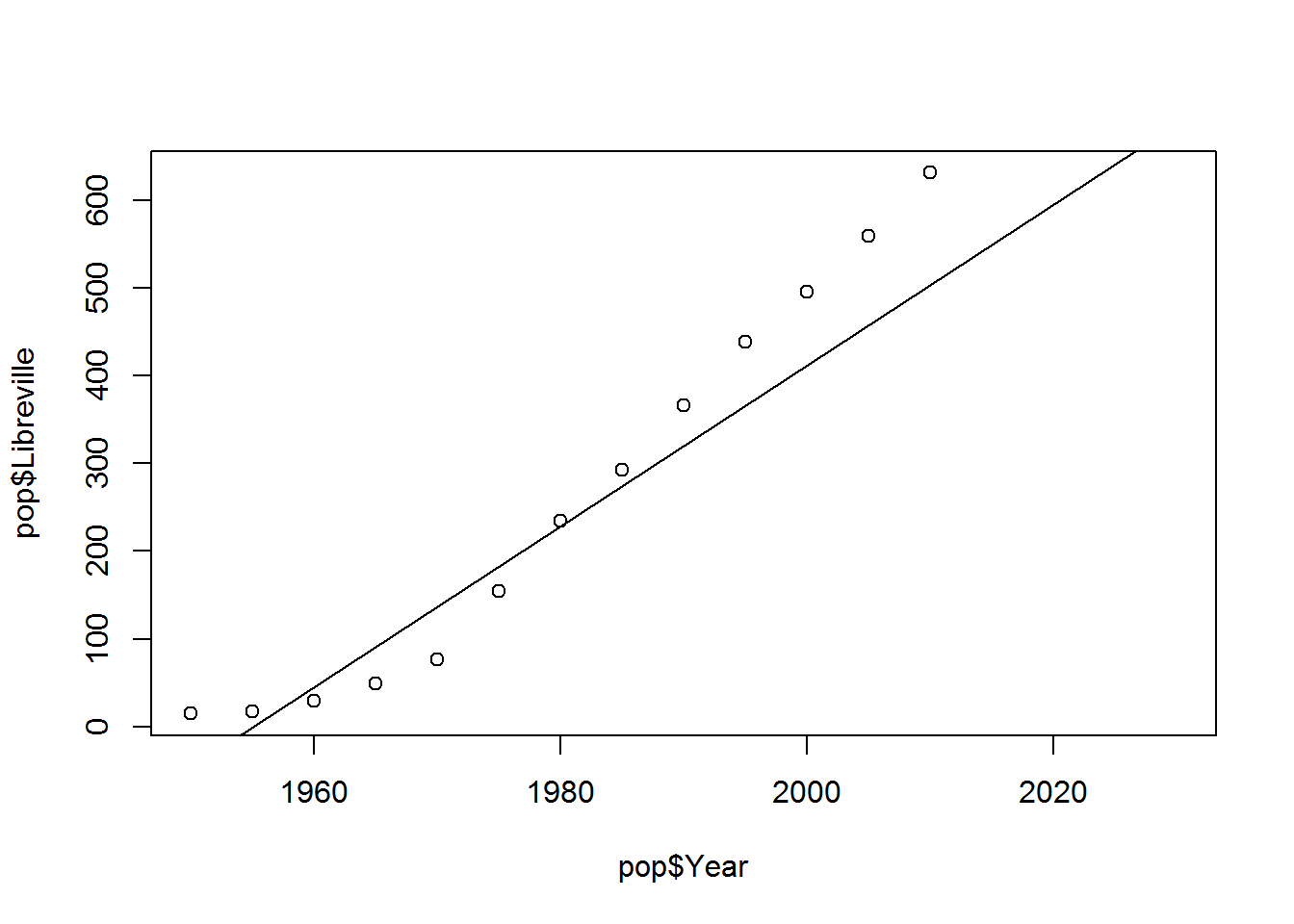This does not look nearly so good. The R2 of lbv_back suggests that we explain 90% of the variance in the data, but the prediction error for the predictor years is much worse. We can compare the mean squared error of the new data to the old data.

``mean(resid(lbv_back)^2)``
``##  1438.128``
``mean((pop\$Libreville[pop\$Year > 1994 & pop\$Year < 2015] - predict(lbv_back, newdata = subset(pop, pop\$Year > 1994 & pop\$Year < 2015)))^2)``
``##  9702.728``

Our average squared prediction error for the 1995 to 2010 is seven times higher than the prediction error between 1955 and 1990. In fact, we would have better predictions of the population from 1995 to 2010, just by taking the average population between 1995 and 2010 and calling it a day.

There are a few ways that we might try to get better predictions.

The data appear to show two distinct trends, one before and one after 1970. We could estimate a regression model using just the more recent data:

``````plot(pop\$Year, pop\$Libreville)
lbv <- lm(Libreville ~ Year, subset(pop, pop\$Year > 1970))
summary(lbv)``````
``````##
## Call:
## lm(formula = Libreville ~ Year, data = subset(pop, pop\$Year >
##     1970))
##
## Residuals:
##     Min      1Q  Median      3Q     Max
## -6.6667 -3.5595 -0.9524  3.5060  8.8095
##
## Coefficients:
##               Estimate Std. Error t value Pr(>|t|)
## (Intercept) -2.636e+04  3.534e+02  -74.58 3.91e-10 ***
## Year         1.343e+01  1.774e-01   75.70 3.58e-10 ***
## ---
## Signif. codes:  0 '***' 0.001 '**' 0.01 '*' 0.05 '.' 0.1 ' ' 1
##
## Residual standard error: 5.747 on 6 degrees of freedom
##   (4 observations deleted due to missingness)
## Multiple R-squared:  0.999,  Adjusted R-squared:  0.9988
## F-statistic:  5731 on 1 and 6 DF,  p-value: 3.576e-10``````
``abline(lbv)``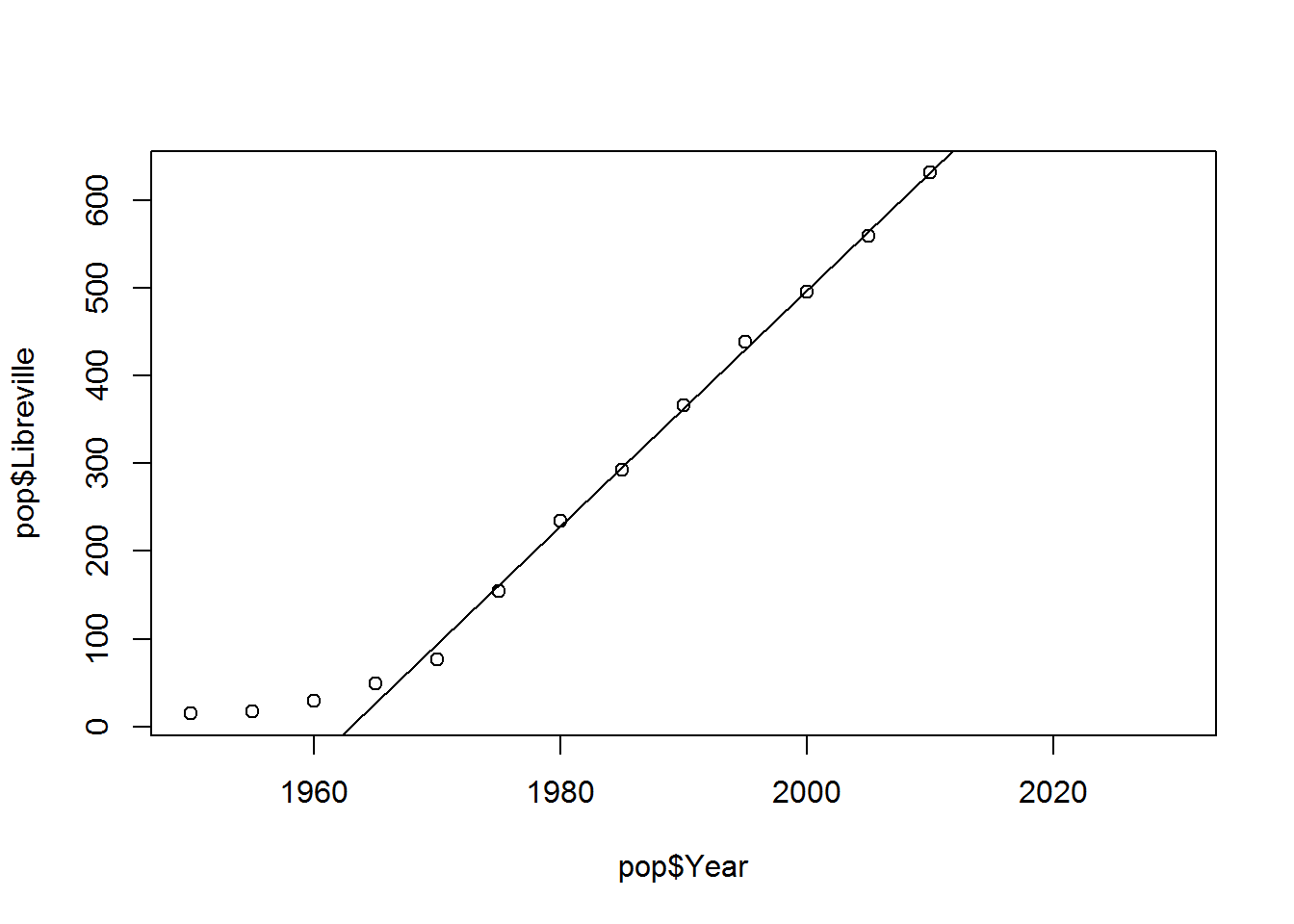Though not necessarily well-suited to these data, adding a quadratic terms can often improve model fit:

``````lbv2 <- lm(pop\$Libreville ~ pop\$Year + I(pop\$Year^2))
summary(lbv2)``````
``````##
## Call:
## lm(formula = pop\$Libreville ~ pop\$Year + I(pop\$Year^2))
##
## Residuals:
##     Min      1Q  Median      3Q     Max
## -41.701 -11.787   6.736  15.260  29.637
##
## Coefficients:
##                 Estimate Std. Error t value Pr(>|t|)
## (Intercept)    4.190e+05  8.743e+04   4.792 0.000733 ***
## pop\$Year      -4.341e+02  8.832e+01  -4.915 0.000609 ***
## I(pop\$Year^2)  1.124e-01  2.230e-02   5.042 0.000505 ***
## ---
## Signif. codes:  0 '***' 0.001 '**' 0.01 '*' 0.05 '.' 0.1 ' ' 1
##
## Residual standard error: 24.95 on 10 degrees of freedom
##   (4 observations deleted due to missingness)
## Multiple R-squared:  0.9894, Adjusted R-squared:  0.9873
## F-statistic: 468.3 on 2 and 10 DF,  p-value: 1.315e-10``````
``````plot(pop\$Year, pop\$Libreville)

lines(pop\$Year[pop\$Year < 2014], predict(lbv2), col=3)``````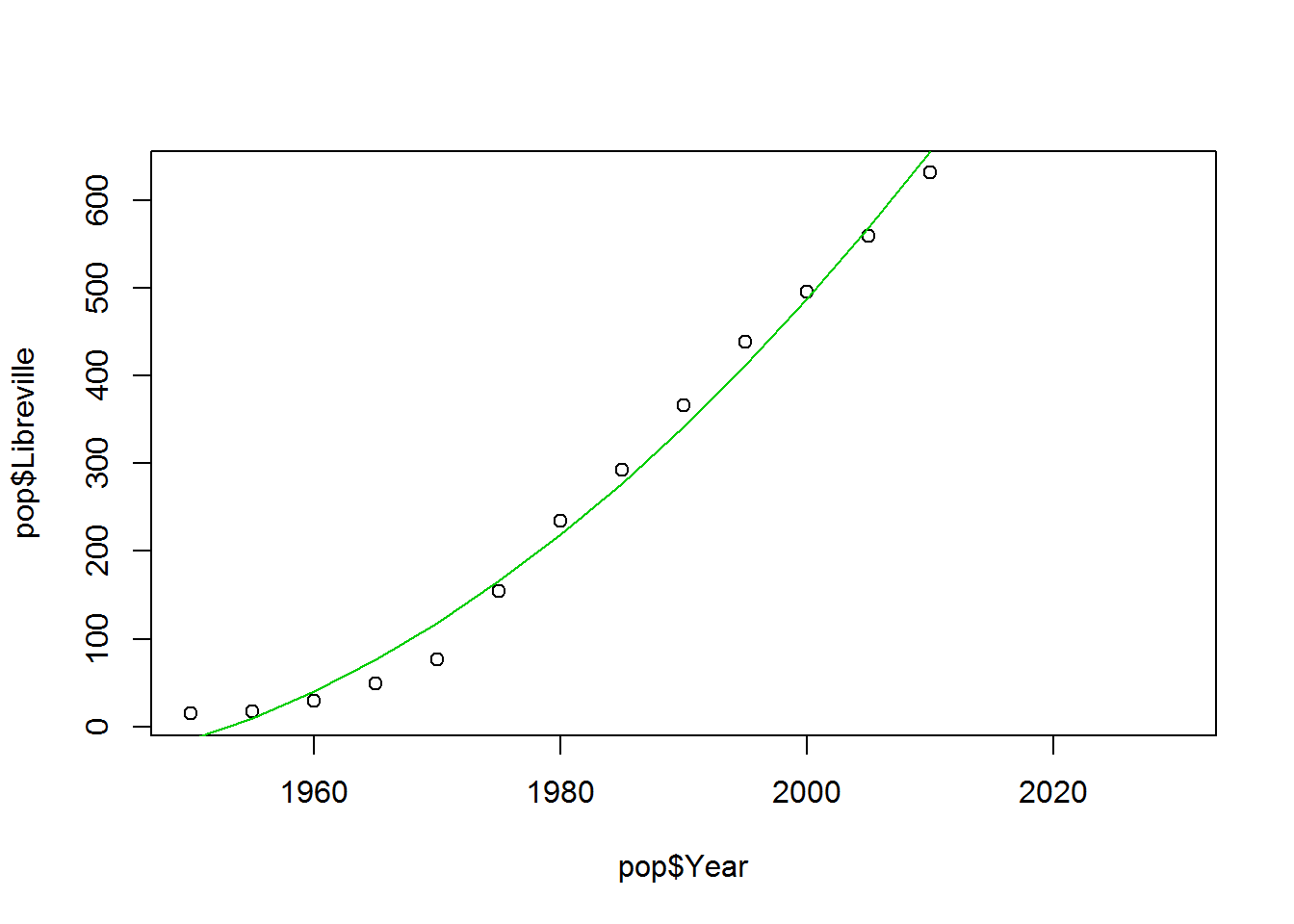Or we could try predicting Libreville’s population as a function of a larger geography, such as all Sub-Saharan African cities over 300,000.

``````plot(pop\$Sub_Saharan_300kplus, pop\$Libreville)

summary(lm(Libreville~Sub_Saharan_300kplus,pop))``````
``````##
## Call:
## lm(formula = Libreville ~ Sub_Saharan_300kplus, data = pop)
##
## Residuals:
##    Min     1Q Median     3Q    Max
## -50.44 -27.04 -23.48  41.54  51.95
##
## Coefficients:
##                       Estimate Std. Error t value Pr(>|t|)
## (Intercept)          22.016224  15.931117   1.382    0.194
## Sub_Saharan_300kplus  0.004803   0.000242  19.850 5.79e-10 ***
## ---
## Signif. codes:  0 '***' 0.001 '**' 0.01 '*' 0.05 '.' 0.1 ' ' 1
##
## Residual standard error: 38.14 on 11 degrees of freedom
##   (4 observations deleted due to missingness)
## Multiple R-squared:  0.9728, Adjusted R-squared:  0.9704
## F-statistic:   394 on 1 and 11 DF,  p-value: 5.795e-10``````
``abline(lm(Libreville~Sub_Saharan_300kplus,pop))``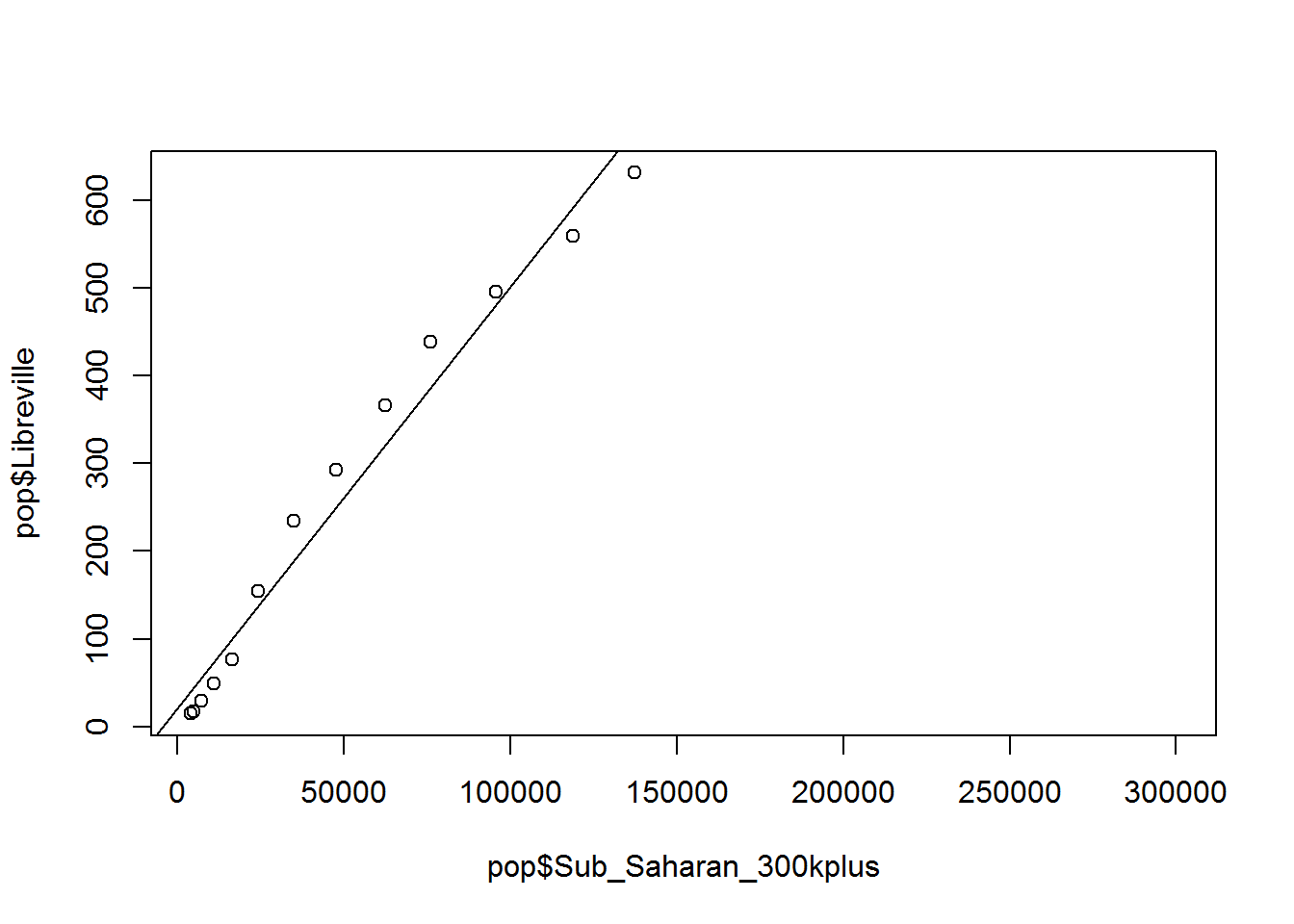Or we could take advantage of the knowledge of the population in the year immediately before our prediction. Our previous models ignored the fact that the 2010 data is a lot more important than any of the other years of data for predicting the population in the future.

First, we need to create a new time-lagged population variable. Walk through the code slowly using the command head(pop_n1) between each line of code to see exactly what you are doing.

``````pop_n1 <- pop[c("Libreville", "Year")]
pop_n1\$Year <- pop_n1\$Year + 5
pop_n1\$Libreville_m5 <- pop_n1\$Libreville
pop_n1 <- pop_n1[c(2:3)]
pop_n1 <- subset(pop_n1, pop_n1\$Year < 2015)
pop <- merge(pop, pop_n1, by = "Year", all.x = T)``````

Now predict Libreville’s population using the population 5 years earlier and the year.

``````lbv3 <- lm(Libreville ~ Libreville_m5 + Year, pop)
summary(lbv3)``````
``````##
## Call:
## lm(formula = Libreville ~ Libreville_m5 + Year, data = pop)
##
## Residuals:
##     Min      1Q  Median      3Q     Max
## -22.877  -6.747  -1.215   8.818  15.653
##
## Coefficients:
##                 Estimate Std. Error t value Pr(>|t|)
## (Intercept)   -9.271e+03  1.961e+03  -4.726  0.00108 **
## Libreville_m5  6.705e-01  9.021e-02   7.432 3.96e-05 ***
## Year           4.740e+00  9.995e-01   4.742  0.00106 **
## ---
## Signif. codes:  0 '***' 0.001 '**' 0.01 '*' 0.05 '.' 0.1 ' ' 1
##
## Residual standard error: 12.79 on 9 degrees of freedom
##   (5 observations deleted due to missingness)
## Multiple R-squared:  0.9972, Adjusted R-squared:  0.9966
## F-statistic:  1601 on 2 and 9 DF,  p-value: 3.271e-12``````

This model explains 99.7% of the variance in the data an indicates that the population in 2015 is equal to the population in 2010 x .67 + 2010 (the year) x 4.74. To estimate the population in 2020, you will need to add the 2015 population estimate.

``````plot(pop\$Year, pop\$Libreville)
lines(pop\$Year[pop\$Year < 2014 & pop\$Year > 1950], predict(lbv3), col=3)``````